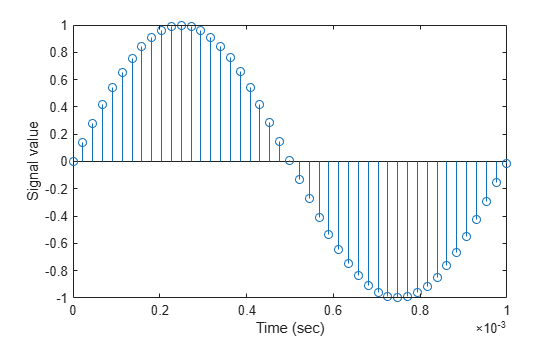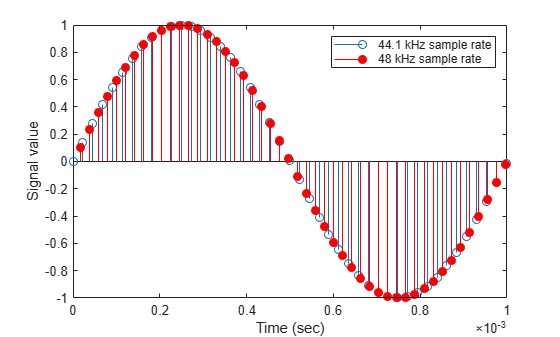# fdesign.polysrc

Construct polynomial sample-rate converter (POLYSRC) filter designer

## Syntax

```d = fdesign.polysrc(l,m) d = fdesign.polysrc(l,m,'Fractional Delay','Np',Np) d = fdesign.polysrc(...,Fs) ```

## Description

`d = fdesign.polysrc(l,m)` constructs a polynomial sample-rate converter filter designer D with an interpolation factor L and a decimation factor M. L defaults to 3. M defaults to 2. L and M can be arbitrary positive numbers.

`d = fdesign.polysrc(l,m,'Fractional Delay','Np',Np)` initializes the filter designer specification with Np and sets the polynomial order to the value Np. If omitted Np defaults to 3.

`d = fdesign.polysrc(...,Fs)` specifies the sampling frequency (in Hz).

## Examples

collapse all

This example shows how to design sample-rate converter that uses a 3rd order Lagrange interpolation filter to convert from 44.1kHz to 48kHz.

```[L,M] = rat(48/44.1); f = fdesign.polysrc(L,M,'Fractional Delay','Np',3); Hm = design(f,'lagrange');```

Original sampling frequency

`Fs = 44.1e3;`

9408 samples, 0.213 seconds long

`n = 0:9407;`

Original signal, sinusoid at 1kHz

`x = sin(2*pi*1e3/Fs*n);`

10241 samples, still 0.213 seconds

`y = filter(Hm,x);`

Plot original sampled at 44.1kHz

```stem(n(1:45)/Fs,x(1:45)) hold on```Plot fractionally interpolated signal (48kHz) in red

```stem((n(3:51)-2)/(Fs*L/M),y(3:51),'r','filled') xlabel('Time (sec)');ylabel('Signal value') legend('44.1 kHz sample rate','48 kHz sample rate')```## Version History

Introduced in R2011a